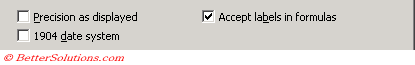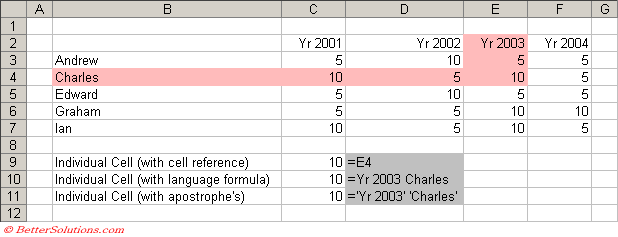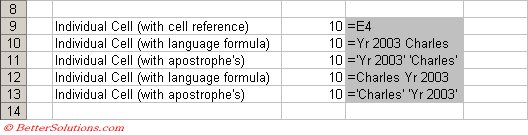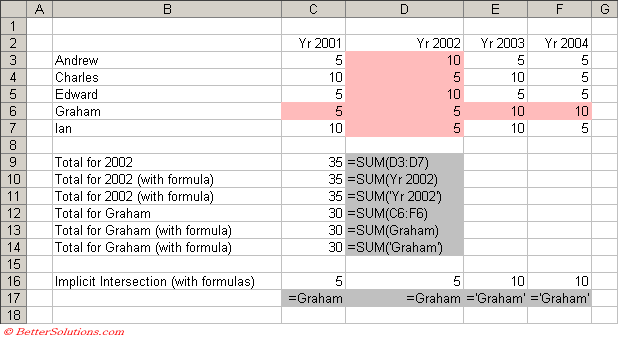# Natural Language Formulas

Removed in 2007
You can use the labels of columns and rows on a worksheet to refer to the cells within those columns and rows.
Labels can only be used in formulas that refer to data on the same worksheet; if you want to represent a range on another worksheet then you must use named range.
This feature is an alternative to using named ranges and is switched off by default.
Natural Language Formulas lets you use column labels and row labels instead of cell references in your formulas.
This can only be used to refer to cells on the same worksheet and can be useful when your table already has column and row labels.
If you want to refer to a cell or range of cells on another worksheet, then you have to use a named range.
When you use text in a formula (instead of a cell reference) Excel will automatically search for any matching column or row labels.
If a match is found then the corresponding cell reference is used. If no match is found then an error is returned.

### Check your (Tools > Options)

Natural Language Formulas are not allowed by default so check your (Tools > Options)(Calculation tab).
This will only work if you have your "Accept labels in formulas" option ticked.
This setting is workbook specific and must be changed in all the necessary workbooks.Any cell references used in new formulas will be automatically replaced with the corresponding labels when this option is checked.
Any existing cell references will be left unchanged.

### Known Problems

To ensure that you do not encounter any problems it is recommended to always enclose your labels in aprostrophe's (').
You may experience some problems using Natural Language Formulas when any of the following are true.
The workbook in which you are entering the formula contains a globally-defined name that is identical to one of the labels in the formula, or the worksheet in which you are entering the formula contains a locally-defined name that is identical to one of the labels in the formula.
One of the labels in the formula is the same as a function that is built into Microsoft Excel.
The workbook in which you are entering the formula contains a reference to another workbook that contains a subroutine or function with a name that is identical to one of the labels in the formula.
The workbook in which you are entering the formula contains a subroutine or function with a name that is identical to one of the labels in the formula.

### Create your table with Labels

You can use the column labels to identify an individual column and the row labels to identify an individual row.
You can therefore use a combination of both column and row labels to identify an individual cell.
The column and row labels in your table can contain spaces.
In the table below the column labels can be found in row "2" and the row headings can be found in column "B".We can obviously refer to individual cells as usual and the formula in cell "C9" is just a normal cell reference to cell "E4".
The formula in cell "C10" uses a natural language formula (i.e. a column label and a row label) to identify the same cell.
The formula in cell "C11" is identical except the labels are enclosed within apostrophe's.
You must enter a space between the row and column labels in the formula as the space character is the intersection operator for cell ranges.
Assuming your (Tools > Options) checkbox is ticked, Excel will automatically try and match your column and row labels if you use any descriptive names in your formulas.

### Is it column then row or row then column ?

Formula labels work on the intersection of both a column and a row.
For this reason it does not matter which way round the labels go, the same cell is always referenced, either column then row or row then column.
Column and then row is the preferred method.
The formula in cell "C10" uses column then row and the formula in cell "C12" uses row and then column.
The formulas is cells "C11" and "C13" are identical except the labels are enclosed within apostrophe's.### Using Complicated Formulas

If a natural language formula is in the same column or row as the column (or row) label it refers to, then the entire row or column is used.
If a natural language formula is in a different column or row than the one it refers to, then the intersection of that row and column is used.
You should always try to use some text in your data labels, as numbers might be treated as numerical arguments as opposed to data labels.
It is even possible to use your table column and row labels as arguments to worksheet functions.The formula in cell "C9" contains the sum of cells "D3:D7" which is equivalent to all the numeric values in the Yr 2002 column of the table.
The formula in cell "C10" refers to the same block of cells in column "E" and is passed to the SUM function.
It is important to know that the formula "=SUM(Yr 2002)" only refers to the data table that contains the labels (i.e. cell range "D3:D7") and not the whole of column "D". This is very different from the formula "SUM(D:D)" which in this case would return 40.
Implicit intersection uses the row or column of the cell containing the formula in order to provide the intersection.
In cell "C16" the formula just refers to a row heading. Therefore the cell that is referenced is the intersection of this row with the column of the cell containing the formula, i.e. column "C". This is identical to an explicit reference to cell C6.
The same formula has been placed in cells D16, E16 and F16.
Because the intersection is implicit the referenced cell is always in the same column as the cell containing the formula.

### Copying your Natural Language Formulas

You can copy and paste these formulas and the cell references will adjust accordingly.
Remember that these formulas use the intersection of columns and rows and also depend on the cell containing the formula.
If you copy the formulas to an area of the worksheet where the formulas do not make sense and the column or row labels are not available then #NAME? will be displayed in the cell.
Changing the names of your column and row labels will result in the formulas changing automatically.

`AcceptLabelsInFormula = True FormulaLabel = xlColumn / RowLabel `

The contents of the cell can be defined to be the name for the row or column.

### Important

Always leave a blank row and column between the data table and any formula referring to it.
You must enter a space between the row and column labels in the formula.
You can enter the references either column and then row (2003 Charles) or by row and then column (Charles 2003). It is more intuitive to use column and then row, although it will probably depend on your data.
If you later change the row and column headings in your table, the formula labels will change automatically.
A formula using labels will return "#NAME?" is it cannot find the corresponding data label.
If you enter a label that is ambiguous Excel will display a Identify Label dialog box.
You can only use formula labels on the same worksheet that the table is on. They will not work on other worksheets.
These types of named ranges cannot be reference from VBA.
Moving or copying a worksheet will automatically replace these with their equivalent cell references.